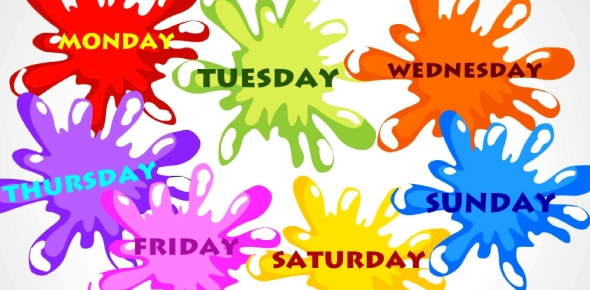# The Real Number System

10 Questions | Total Attempts: 71SettingsBell Work for Algebra I Monday September 16, 2013

Related Topics
• 1.
What is the sum of 25 and its opposite?
• A.

-50

• B.

0

• C.

25

• D.

50

• 2.
Which of the following is NOT a rational number?
• A.

-(30/6)

• B.

63/5

• C.

9.8

• D.

3.456789120

• 3.
Simplify:
• A.

10

• B.

23

• C.

50

• D.

54

• 4.
Name the property that is applied in this problem:  (72 + 5) + 16 = 72 + ( 5 + 16)
• A.

Commutative Property

• B.

Associative Property

• C.

Distributive Property

• D.

• 5.
What property is shown in this problem:  5(2 + 15) = (5)(2) + (5)(15)?
• A.

Commutative Property

• B.

Associative Property

• C.

Distributive Property

• D.

Multiplicative identity

• 6.
What property is illustrated by:  (35)(45) = 1575      (45)(35) = 1575
• A.

Commutative Property

• B.

Associative Property

• C.

Distributive Property

• D.

• 7.
Simplify:
• A.

468

• B.

234

• C.

0

• D.

-234

• 8.
Simplify:  -
• A.

45

• B.

-45

• C.

0

• D.

90

• 9.
What is the reciprocal of -3/2?
• A.

3/2

• B.

2/3

• C.

-2/3

• D.

6

• 10.
The product of a number and its reciprocal always equals one
• A.

True

• B.

False